GeeksforGeeks App
Open AppBrowser
Continue

## Related Articles

• RD Sharma Class 10 Solutions

# Class 10 RD Sharma Solutions – Chapter 5 Trigonometric Ratios – Exercise 5.1 | Set 3

### Question 22. If sinθ = a/b, find secθ + tanθ in terms of a and b.

Solution:

Given:

sinθ = a/b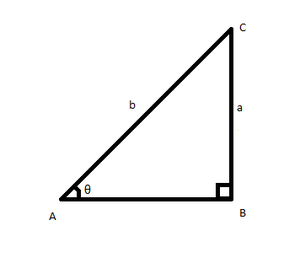From Pythagoras theorem,

AC2 = BC2 + AB2

b2 = a2 + AB2

AB2=Now,

= secθ + tanθ

=====### Question 23. If 8tanA = 15, find sin A − cos A.

Solution:

Given:

8tanA = 15

tanA = 15/8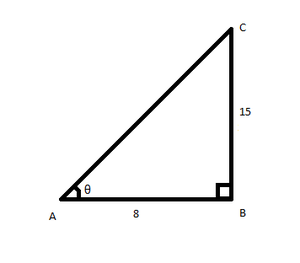From pythagoras theorem,

AC2 = BC2 + AB2

AC2 = 152 + 82

AC2 = 225 + 64 = 289

AC = 17

Now,

= sin A − cos A

= 15/17 – 8/17

= (15 – 8)/17

= 7/17

### Question 24. If tanθ = 20/21, show that.

Solution:

Given: tanθ = 20/21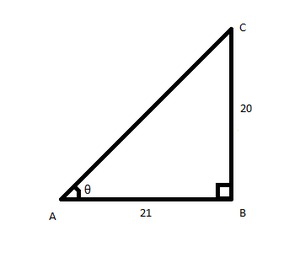From Pythagoras theorem,

AC2 = BC2 + AB2

AC2 = 202 + 212

AC2 = 400 + 441 = 841

AC = 29

Now, taking LHS

==== 30/70

= 3/7

### Question 25. If cosec A = 2, find the value of.

Solution:

Given:

cosec A = 2

We know

sin A = 1/cosecA = 1/2

And, sin 30° = 1/2

A = 30°

tan30° = 1/√3 and cos30° = √3/2

Now,

======= 2

### Question 26. If ∠A and ∠B are acute angles such that cos A = cos B, then show that ∠A = ∠B.

Solution:

Let us consider a △ABC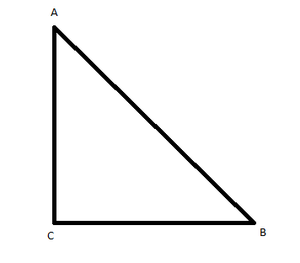From the figure,

Given,

cos A = cos B

AC/AB = BC/AB

Multiplying both side by AB

(AC/AB) × AB = (BC/AB) × AB

AC = BC

In △ABC, AC = BC So we can say that the triangle is an isosceles triangle,

and in an isosceles triangle we know that if two sides of a triangle are equal,

then the angle opposite to the sides are equal.

Therefore, ∠A =∠B

### Question 27. In a Δ ABC, right angled at A, if tanC = √3, find the value of sin B cos C + cos B sin C.

Solution:

In right angled Δ ABC,

Given: tan C = √3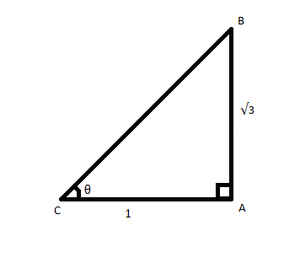∴AB = √3 and AC = 1

From pythagoras theorem,

BC2 = AB2 + AC2

BC2 = (√3)2 + 12

BC2 = 3 + 1 = 4

BC = 2

Therefore,

sin B cos C + cos B sin C

= (1/2)(√3/2) + (√3/2)(√3/2)

= 1/4 + 3/4

= 4/4

= 1

### (i) The value of tan A is always less than 1.

Solution:

FALSE. The value of tan A is not always less than 1.

Consider the Pythagorean triplet, 13, 12, and 5

where, 13 is the hypotenuse

We know

tan A = Perpendicular/Base

Let Perpendicular = 12 and Base = 5

then, tanA = 12/5 = 2.4 which is greater than 1.

### (ii) sec A = 12/5 for some value of angle A.

Solution:

TRUE

We have sec A = 12/5 for some value of ∠A

secθ = Hypotenuse/Base

In a right angled triangle, hypotenuse is the greatest side.

So secθ > 1 is valid

Here, secθ = 12/5

### (iii) cos A is the abbreviation used for the cosecant of angle A.

Solution:

FALSE

cos A means cosine of ∠A

cos A = Base/Hypotenuse

However,

cosec A = Hypotenuse/Perpendicular

### (iv) cot A is the product of cot and A.

Solution:

FALSE

cot A means Cotangent of ∠A

cot A = 1/tanA

Only “cot” doesn’t defines anything.

Hence, cot A is not the product of cot and A.

### (v)  sinθ = 4/3 for some angle θ.

Solution:

FALSE

sinθ = 4/3 for some value of ∠θ

We have,

sinθ = Perpendicular/Hypotenuse

In a right angled triangle, hypotenuse is the greatest side.

So sinθ is always less than 1.

Here, sinθ = 4/3 = 1.3 which is greater than 1

### Question 29. If sinθ = 12/13, find the value of.

Solution:

Given:

sinθ = 12/13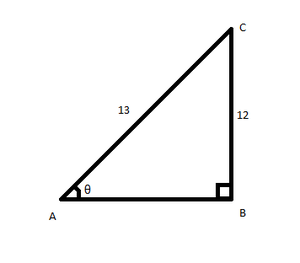Using Pythagoras theorem,

AC2 = BC2 + AB2

132 = 122 + AB2

AB2 = 169 − 144 = 25

AB = 5===== 595/3456

### Question 30. If cosθ = 5/13, find the value of.

Solution:

Given:

cosθ = 5/13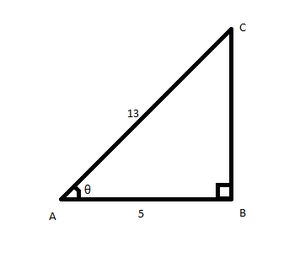Using Pythagoras theorem,

AC2 = BC2 + AB2

132 = BC2 + 52

BC2 = 169 − 25 = 144

BC = 12=== 595/3456

### Question 31. If secA = 5/4, verify that.

Solution:

Given:

secA = 5/4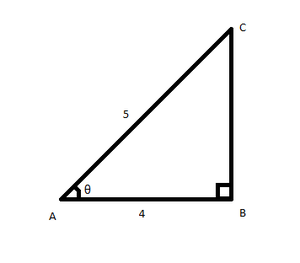From pythagoras theorem,

AC2 = BC2 + AB2

52 = BC2 + 42

BC2 = 25 − 16 = 9

BC = 3

Now

====== 117/-44 = 117/(11(4))

= 117/-44 = 117/-44

Hence Proved

### Question 32. If sinθ = 3/4, prove that.

Solution:

Given: sinθ = 3/4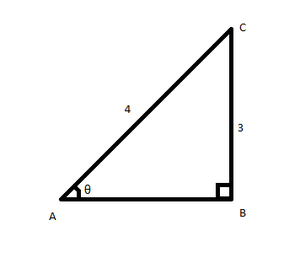From Pythagoras theorem,

AC2 = BC2 + AB2

42 = AB2 + 32

AB2 = 16 – 9 = 7

AB =√7

We have,Now squaring both side

=== 7/9

We know

1 + cot2θ = cosec2θ

1 + tan2θ = sec2θ

= 1/tan2θ = 7/9

== 7/9 = 7/9

Hence Proved

### Question 33. If secA = 17/8, verify that.

Solution:

Given: secA = 17/8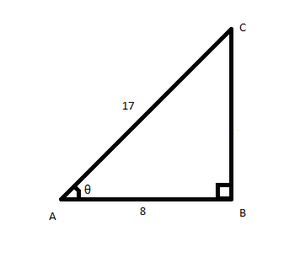From Pythagoras theorem,

AC2 = BC2 + AB2

172 = BC2 + 82

BC2 = 289 − 64 = 225

BC = 15We havePutting the values of sinA, cosA and tanA in the above equation

===== 33/611 = 33/611

Hence Proved

### Question 34. If cotθ = 3/4, prove that.

Solution:

Given:  cotθ = 3/4

tanθ = 4/3

Using the pythagoras theorem

sinθ = 4/5, cosθ = 3/5

cosecθ = 5/4, secθ = 5/3

Now, taking LHS

===== 1/√7

### Question 35. If 3cosθ − 4sinθ = 2cosθ + sinθ, then find tanθ.

Solution:

Given: 3cosθ − 4sinθ = 2cosθ + sinθ

Dividing both equation by cosθ we get,3 – 4tanθ = 2 + tanθ

3 – 2 = 4tanθ + tanθ

tanθ = 1/5

### Question 36. If ∠A and ∠B are acute angles such that tan A = tan B, then show that ∠A = ∠B.

Solution:

Let us consider a △ABCFrom the figure,

Given:

tan A = tan B

BC/AC = AC/BC

AC2 = BC2

AC = BC

In △ABC, AC = BC So we can say that the triangle is an isosceles triangle, √3

and in an isosceles triangle we know that if two sides of a triangle are equal,

then the angle opposite to the sides are equal.

Therefore ∠A =∠B

My Personal Notes arrow_drop_up
Related Tutorials